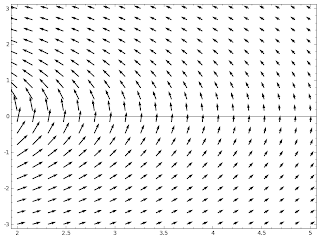## A example where Green's theorem does not applyplot_vector_field((-y/(x^2 + y^2), x/(x^2 + y^2)), (x, 2, 5), (y, -3, 3))


A example where Green's theorem does not apply

Suppose we have a force field (plotted in the figure) \begin{align*} F &= \begin{pmatrix} -y / (x^2 + y^2) \\ x / (x^2 + y^2) \end{pmatrix} \end{align*} You can check that $\text{curl }F= 0$ everywhere except at the origin, where F is not defined. Suppose an object moves in F in a unit circle, then $x^2 + y^2 = 1$, and F simplifies to \begin{align*} F &= \begin{pmatrix} -y \\ x \end{pmatrix} \end{align*} Then if you parameterize with $x = \cos\theta \cdots$, you can easily get the work done is $2\pi$. But if you use Green's theorem, \begin{align*} \int_c F\cdot dr &= \iint_R \text{curl } F dA \\ &= 0 \end{align*} Clearly it's wrong here, because one assumption of Green's theorem is violated: F must be defined everywhere in the region.